Homework Help Question & Answers

# CH(CH),CH) will react with gaseous oxypen (0) to produce gaseous carbon dioxide (C0.) and gaseous water...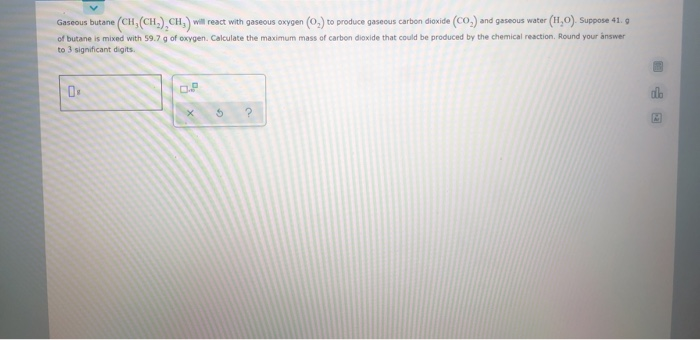CH(CH),CH) will react with gaseous oxypen (0) to produce gaseous carbon dioxide (C0.) and gaseous water (H,0). Suppose 41.9 Gaseous butane of butane is mixed with 59.7 g of oxygen. Calculate the maximum mass of carbon dioxide that could be produced by the chemical reaction. Round your answer to 3 significant digits. 5 ?

#### Homework Answers

Answer #1

2C4H10 + 13O2 = 8CO2 + 10H2O

number of moles of butane = 41/58 = 0.71 moles

number of moles of oxygen = 59.7/32 = 1.86 moles

for 1 mole of butane we need 5.5 moles of oxygen

1.86/5.5 = 0.34

we have only enough oxygen to react with 0.34 moles of butane

oxygen is the limiting reagent

number of moles of CO2 = 4 x 0.34 = 1.36 moles

mass of CO2 =1.36 x 44 =59.84 g

Know the answer?
Your Answer:

#### Post as a guest

Your Name:

What's your source?

#### Earn Coin

Coins can be redeemed for fabulous gifts.

Not the answer you're looking for? Ask your own homework help question. Our experts will answer your question WITHIN MINUTES for Free.
Similar Homework Help Questions
• ### Gaseous butane (CH(CH), CH) will react with gaseous oxygen (0,) to produce gaseous carbon dioxide (CO)...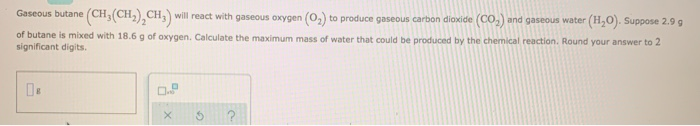Gaseous butane (CH(CH), CH) will react with gaseous oxygen (0,) to produce gaseous carbon dioxide (CO) and gaseous water (H2O). Suppose 2.99 of butane is mixed with 18.6 g of oxygen. Calculate the maximum mass of water that could be produced by the chemical reaction. Round your answer to 2 significant digits. X 5 ?

• ### Gaseous butane (CH,(CH), CH,) will react with gaseous oxygen (0.) to produce gaseous carbon dioxide (CO) and gaseou...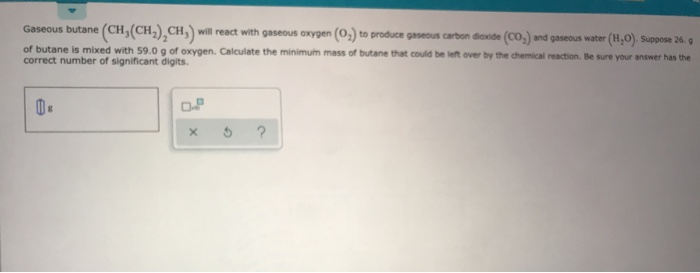Gaseous butane (CH,(CH), CH,) will react with gaseous oxygen (0.) to produce gaseous carbon dioxide (CO) and gaseous water (1,0). Suppose 26. of butane is mixed with 59.0 g of oxygen. Calculate the minimum mass of butane that could be left over by the chemical reaction. Be sure your answer has the correct number of significant digits.

• ### Gaseous ethane (CH,CH,) will react with gaseous oxygen (0) to produce gaseous carbon dioxide (CO) and gaseous water...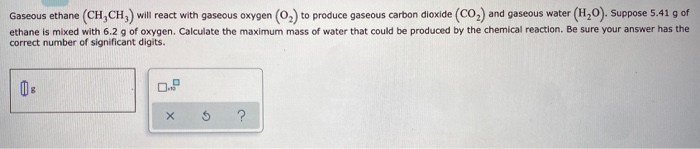Gaseous ethane (CH,CH,) will react with gaseous oxygen (0) to produce gaseous carbon dioxide (CO) and gaseous water (HO). Suppose 5.41 g of ethane is mixed with 6.2 g of oxygen. Calculate the maximum mass of water that could be produced by the chemical reaction. Be sure your answer has the correct number of significant digits. x 5 ?

• ### Liquid octane (CH,(CH), CH,) will react with gaseous oxygen (O2) to produce gaseous carbon dioxide (CO,)...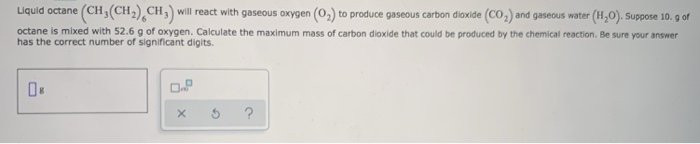Liquid octane (CH,(CH), CH,) will react with gaseous oxygen (O2) to produce gaseous carbon dioxide (CO,) and gaseous water (H,0). Suppose 10. g of octane is mixed with 52.6 g of oxygen. Calculate the maximum mass of carbon dioxide that could be produced by the chemical reaction. Be sure your answer has the correct number of significant digits. xs ?

• ### (сн, сH), сн-) (CH will react with gaseous oxygen (0, to produce gaseous carbon dioxide CO2...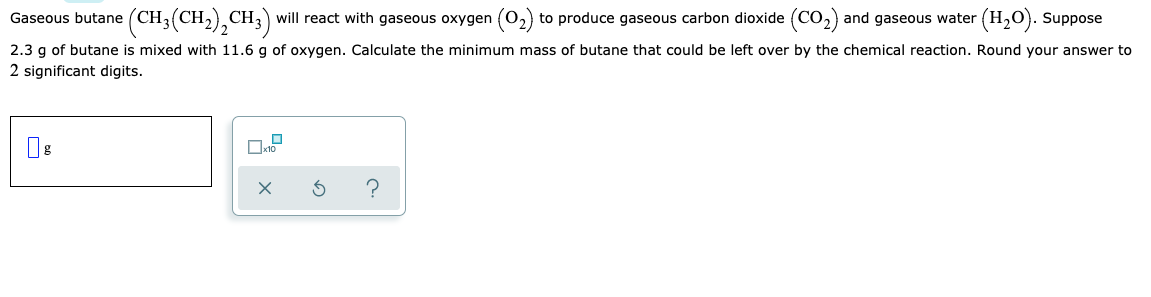(сн, сH), сн-) (CH will react with gaseous oxygen (0, to produce gaseous carbon dioxide CO2 and gaseous water (H,O). Suppose Gaseous butane 2.3 q of butane is mixed with 11.6 q of oxyqen. Calculate the minimum mass of butane that could be left over by the chemical reaction. Round your answer to 2 significant digits. ? X

• ### Gaseous methane (CH) will react with gaseous oxygen (O2) to produce gaseous carbon dioxide (CO) and...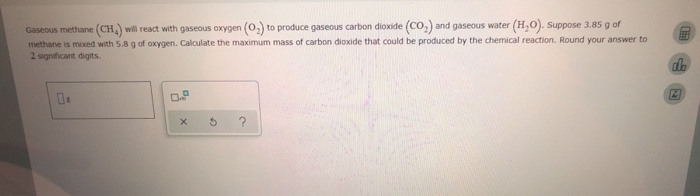Gaseous methane (CH) will react with gaseous oxygen (O2) to produce gaseous carbon dioxide (CO) and gaseous water methane is mixed with 5.8 g of oxygen. Calculate the maximum mass of carbon dioxide that could be produced by the chemical reaction. Round your answer to 2 significant digits. (H,O). Suppose 3.85 g of

• ### O STOICHOMETRY Limiting reactants Gaseous butane (CH(CH), CH) will react with gaseous oxygen (0) to produce...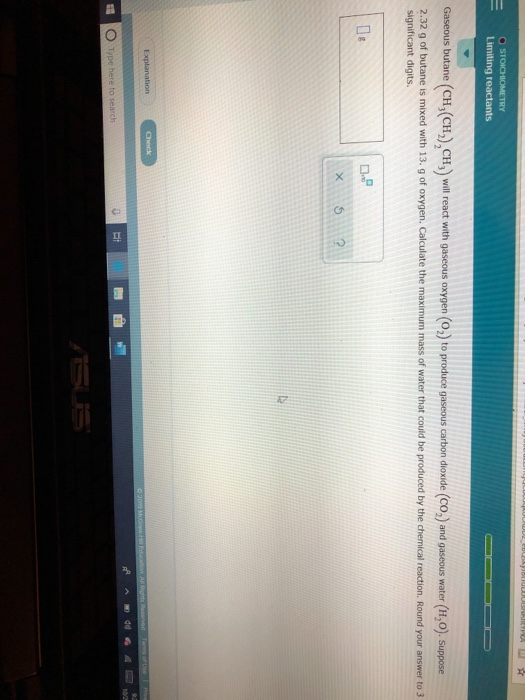O STOICHOMETRY Limiting reactants Gaseous butane (CH(CH), CH) will react with gaseous oxygen (0) to produce gaseous carbon dioxide (CO) and gaseous water (H,0). Suppose 2.32 g of butane is mixed with 13. g of oxygen. Calculate the maximum mass of water that could be produced by the chemical reaction. Round your answer to 3 significant digits. De Explanation Ock O Type here to search

• ### Gaseous ethane (CH,CH) will react with gaseous oxygen (02) to produce gaseous carbon dioxide (CO) and...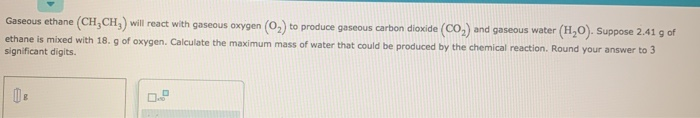Gaseous ethane (CH,CH) will react with gaseous oxygen (02) to produce gaseous carbon dioxide (CO) and gaseous water (H,O). Suppose 2.41 g of ethane is mixed with 18. g of oxygen. Calculate the maximum mass of water that could be produced by the chemical reaction. Round your answer to 3 significant digits.

• ### (CH, (CH,) CH,) Liquid octane will react with gaseous oxygen (O2) to produce gaseous carbon dioxide...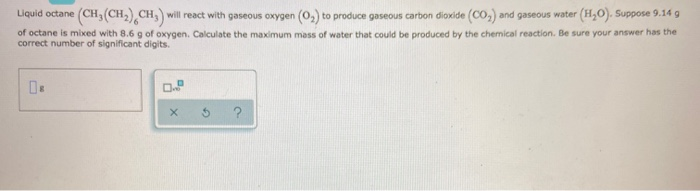(CH, (CH,) CH,) Liquid octane will react with gaseous oxygen (O2) to produce gaseous carbon dioxide (CO2) and gaseous water (H,O). Suppose 9.14 g of octane is mixed with 8.6 g of oxygen. Calculate the maximum mass of water that could be produced by the chemical reaction. Be sure your answer has the correct number of significant digits X

• ### Gaseous butane CH3CH22CH3 will react with gaseous oxygen O2 to produce gaseous carbon dioxide CO2 and...

Gaseous butane CH3CH22CH3 will react with gaseous oxygen O2 to produce gaseous carbon dioxide CO2 and gaseous water H2O. Suppose 26.2 g of butane is mixed with 36. g of oxygen. Calculate the maximum mass of carbon dioxide that could be produced by the chemical reaction. Be sure your answer has the correct number of significant digits.